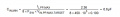# Boost input capacitor calculation

#### BitsNBytes

Joined Mar 22, 2021
35
Hello Members,

I have rarely seen any boost application note that derives the boost input capacitor value. You always see this on the buck output capacitor. For example, a buck output capacitor calculation to first order ideal:

$Cout_{BUCK} = \frac{\Delta I_{L}}{(8*\Delta V_{OUT}*F_{SW})}$

Wouldn't the calculation for CIN for a Boost "simply" be:

$Cin_{Boost} = \frac{\Delta I_{L}}{(8*\Delta V_{IN}*F_{SW})}$

The only application note I have seen to back this up is AN4501 from ST Micro. Page 11:#### BitsNBytes

Joined Mar 22, 2021
35
Well, ok. Then this must be accurate!

#### MrAl

Joined Jun 17, 2014
10,884
Hello Members,

I have rarely seen any boost application note that derives the boost input capacitor value. You always see this on the buck output capacitor. For example, a buck output capacitor calculation to first order ideal:

$Cout_{BUCK} = \frac{\Delta I_{L}}{(8*\Delta V_{OUT}*F_{SW})}$

Wouldn't the calculation for CIN for a Boost "simply" be:

$Cin_{Boost} = \frac{\Delta I_{L}}{(8*\Delta V_{IN}*F_{SW})}$

The only application note I have seen to back this up is AN4501 from ST Micro. Page 11:
View attachment 273981
Hello there,

We could develop a comprehensive formula based on the operation of a boost circuit which typically takes in a lot of current in exchange for a higher output voltage.
That higher input current has to come from the cap for the most part because it's close to the circuit and there will always be some line impedance between the circuit and the power source.
The rating will depend mostly on the input/output voltage ratio as well as the load current and the frequency or time period. It will also depend on the worst case duty cycle.

One thing we really do see more rare is a formula for the current rating of the capacitors. The ripple current rating has to match the expected ripple current caused by the converter. If it doesnt, the cap can overheat and explode. In high power converters this is a major concern and often multiple caps are connected in close parallel to help with that.